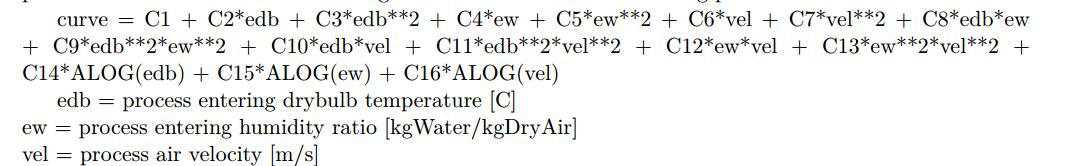Question-and-Answer Resource for the Building Energy Modeling Community
Get s tarted with the Help page

# where is the mathematical model of Dehumidifier:Desiccant:NoFansHello everyone, i have some difficulty to find the mathematical model of the object Dehumidifier:Desiccant:NoFans contained in EnergyPlus, even i have went through the documents of "InputOutputReference" and "EngineeringReference". As specified in the document of "InputOutputReference" , the object of Dehumidifier:Desiccant:NoFans allows the users to use either the default performance model or user specified performance model. As i currently i have no user specified model, so i have to use the default performance model.The default performance model is defined in the "InputOutputReference" by a performance curve of the following form:. This equation includes many coefficients (i.e. C1, C2,C3 and C15), but the values of these coeffieicnts are not given.
Is there anyone can give me some hints to the values of these coefficients. I quite appreciate if anyone can give me some feedbacks. Thank you in advance.

edit retag close merge delete

Sort by » oldest newest most voted

You can find the hardcoded coefficients in the source code.

You'll notice that the hardcoded coefficients are 0-indexed while the engineering reference is 1-indexed, so offset it by 1...

// Calculate leaving conditions
TC0 = -38.7782841989449;
TC1 = 2.0127655837628;
TC2 = 5212.49360216097;
TC3 = 15.2362536782665;
TC4 = -80.4910419759181;
TC5 = -0.105014122001509;
TC6 = -229.668673645144;
TC7 = -0.015424703743461;
TC8 = -69440.0689831847;
TC9 = -1.6686064694322;
TC10 = 38.5855718977592;
TC11 = 0.000196395381206009;
TC12 = 386.179386548324;
TC13 = -0.801959614172614;
TC14 = -3.33080986818745;
TC15 = -15.2034386065714;

ProcAirOutTemp = TC0 + TC1 * ProcAirInTemp + TC2 * ProcAirInHumRat + TC3 * ProcAirVel + TC4 * ProcAirInTemp * ProcAirInHumRat +
TC5 * ProcAirInTemp * ProcAirVel + TC6 * ProcAirInHumRat * ProcAirVel + TC7 * ProcAirInTemp * ProcAirInTemp +
TC8 * ProcAirInHumRat * ProcAirInHumRat + TC9 * ProcAirVel * ProcAirVel +
TC10 * ProcAirInTemp * ProcAirInTemp * ProcAirInHumRat * ProcAirInHumRat +
TC11 * ProcAirInTemp * ProcAirInTemp * ProcAirVel * ProcAirVel +
TC12 * ProcAirInHumRat * ProcAirInHumRat * ProcAirVel * ProcAirVel + TC13 * std::log(ProcAirInTemp) +
TC14 * std::log(ProcAirInHumRat) + TC15 * std::log(ProcAirVel);

more